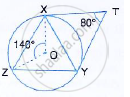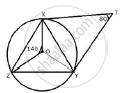Share

# In the Figure, Given Below, O is the Centre of the Circumcircle of Triangle Xyz. Tangents at X and Y Intersect at Point T. Given ∠Xty = 80°, and ∠Xoz = 140°, Calculate The Value of ∠Zxy. - Mathematics

Course

#### Question

In the figure, given below, O is the centre of the circumcircle of triangle XYZ.Tangents at X and Y intersect at point T. Given ∠XTY = 80°, and ∠XOZ = 140°, calculate the  value of ∠ZXY.

#### SolutionIn the figure, a circle with centre O, is the circum circle of triangle XYZ.
∠XOZ =140°
Tangents at X and Y intersect at point T, such that ∠XTY = 80°
∴ ∠XOY = 180° - 80° =100°
But, ∠XOY+ ∠YOZ +∠ZOX = 360° [Angles at a point]
⇒ 100° + ∠YOZ +140° = 360°
⇒ 240°  + ∠YOZ = 360°
⇒ ∠YOZ = 360° - 240°
⇒  ∠YOZ  = 120
Now arc YZ subtends ∠YOZat the centre and ∠YXZat the remaining part of the circle.
∴  ∠YOZ = 2∠YXZ
 ⇒ ∠YXZ =1/2 ∠YOZ
⇒  ∠YXZ  = 1/2 xx120° =  60°

Is there an error in this question or solution?